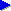Search this siteJava for beginners (topic index)Variablesfor (loops)ifelselogical operatorsarraysobjects# if statements

On the previous page, we looked at Java for lops. The for loop is very important because it is an example of flow control: it allows our program to do something other than just "start at the beginning and blindly go to the end". Pretty much any program beyond the simple Hello World! example needs to control its flow.

Another important type of flow control is the if statement. This allows us to execute a particular section (block) of code if (and only if) a particular condition is true. The if statement is important for allowing our program to make decisions.

As an example, let's say that we want to print out the times tables between 2 and 12 as in our nested loops example. But let's say that we don't want to bother with the 10 times table, because that's easy. We could write two separate loops, one for tables 2-9 and one fo tables 11-12. But that would be a bit clumsy: we'd have to write twice a piece of code that essentially "does the same thing". So instead, we can introduce an if statement to print out the times table on each loop, only if the times table number isn't 10:

```for (int timesTableNo = 2; timesTableNo <= 12; timesTableNo++) {
if (timesTableNo != 10) {
System.out.println("The " + timesTableNo + " times table:");

for (int n = 1; n <= 12; n++) {
int result = n * timesTableNo;
System.out.println(timesTableNo + " times " + n + " = " + n);
}
}
}
```

Notice that the word if is followed by a condition in brackets. As with the for statement, the if statement is followed by a block inside curly braces { ... }. Remember, we said that in general, when you want several lines of code to function together as a "section" of program, you put curly braces around them.

The condition itself is is not equal to, which in Java we write as !=. In the for loop, we actually saw another condition: <= meaning is less than or equal to. Here are common conditions that we can use:

ConditionJava code
is equal to
`if (a == b) {}`
is not equal to
`if (a != b) {}`
is less than
`if (a < b) {}`
is more than
`if (a > b) {}`
is less than or equal to
`if (a <= b) {}`
is more than or equal to
`if (a >= b) {}`

For example, in a card game where we need to check if there are any cards left in the deck, we could write:

```if (noCardsLeft > 0) {
... give card to player ...
}
```

## Next: if ... else

On the next page, we look at how to use the if ... else construct to make a certain block of code run if the condition isn't true.